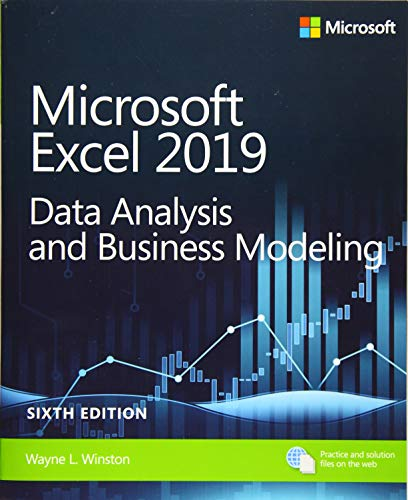# Microsoft Excel 2019 Data Analysis and Business Modeling, 6th Edition

• Length: 880 pages
• Edition: 6
• Language: English
• Publisher:
• Publication Date: 2019-04-25
• ISBN-10: 1509305882
• ISBN-13: 9781509305889
4.4

## Book Description

Master business modeling and analysis techniques with Microsoft Excel 2019 and Office 365 and transform data into bottom-line results. Written by award-winning educator Wayne Winston, this hands-on, scenario-focused guide helps you use Excel to ask the right questions and get accurate, actionable answers. New coverage ranges from Power Query/Get & Transform to Office 365 Geography and Stock data types. Practice with more than 800 problems, many based on actual challenges faced by working analysts.

• Quickly transition from Excel basics to sophisticated analytics
• Use PowerQuery or Get & Transform to connect, combine, and refine data sources
• Leverage Office 365’s new Geography and Stock data types and six new functions
•  Illuminate insights from geographic and temporal data with 3D Maps
• Summarize data with pivot tables, descriptive statistics, histograms, and Pareto charts
• Use Excel trend curves, multiple regression, and exponential smoothing
• Delve into key financial, statistical, and time functions
• Master all of Excel’s great charts
• Quickly create forecasts from historical time-based data
• Use Solver to optimize product mix, logistics, work schedules, and investments—and even rate sports teams
• Run Monte Carlo simulations on stock prices and bidding models
• Learn about basic probability and Bayes’ Theorem
• Use the Data Model and Power Pivot to effectively build and use relational data sources inside an Excel workbook
• Automate repetitive analytics tasks by using macros

Chapter 1. Basic worksheet modeling
Chapter 2. Range names
Chapter 3. Lookup functions
Chapter 4. The INDEX function
Chapter 5. The MATCH function
Chapter 6. Text functions and Flash Fill
Chapter 7. Dates and date functions
Chapter 8. NPV and XNPV functions
Chapter 9. IRR, XIRR, and MIRR functions
Chapter 10. More Excel financial functions
Chapter 11. Circular references
Chapter 12. IF, IFERROR, IFS, CHOOSE, and SWITCH functions
Chapter 13. Time and time functions
Chapter 14. The Paste Special command
Chapter 15. Three-dimensional formulas and hyperlinks
Chapter 16. The auditing tool and the Inquire add-in
Chapter 17. Sensitivity analysis with data tables
Chapter 18. The Goal Seek command
Chapter 19. Using the Scenario Manager for sensitivity analysis
Chapter 20. The COUNTIF, COUNTIFS, COUNT, COUNTA, and COUNTBLANK functions
Chapter 21. The SUMIF, AVERAGEIF, SUMIFS, AVERAGEIFS, MAXIFS, and MINIFS functions
Chapter 22. The OFFSET function
Chapter 23. The INDIRECT function
Chapter 24. Conditional formatting
Chapter 25. Sorting in Excel
Chapter 26. Excel tables and table slicers
Chapter 27. Spin buttons, scrollbars, option buttons, check boxes, combo boxes, and group list boxes
Chapter 28. The analytics revolution
Chapter 29. An introduction to optimization with Excel Solver
Chapter 30. Using Solver to determine the optimal product mix
Chapter 31. Using Solver to schedule your workforce
Chapter 32. Using Solver to solve transportation or distribution problems
Chapter 33. Using Solver for capital budgeting
Chapter 34. Using Solver for financial planning
Chapter 35. Using Solver to rate sports teams
Chapter 36. Warehouse location and the GRG Multistart and Evolutionary Solver engines
Chapter 37. Penalties and the Evolutionary Solver
Chapter 38. The traveling salesperson problem
Chapter 39. Importing data from a text file or document
Chapter 40. Get & Transform
Chapter 41. Geography and Stock data types
Chapter 42. Validating data
Chapter 43. Summarizing data by using histograms and Pareto charts
Chapter 44. Summarizing data by using descriptive statistics
Chapter 45. Using pivot tables and slicers to describe data
Chapter 46. The Data Model
Chapter 47. Power Pivot
Chapter 48. Filled and 3D Power Maps
Chapter 49. Sparklines
Chapter 50. Summarizing data with database statistical functions
Chapter 51. Filtering data and removing duplicates
Chapter 52. Consolidating data
Chapter 53. Creating subtotals
Chapter 54. Charting tricks
Chapter 55. Estimating straight-line relationships
Chapter 56. Modeling exponential growth
Chapter 57. The power curve
Chapter 58. Using correlations to summarize relationships
Chapter 59. Introduction to multiple regression
Chapter 60. Incorporating qualitative factors into multiple regression
Chapter 61. Modeling nonlinearities and interactions
Chapter 62. Analysis of variance: One-way ANOVA
Chapter 63. Randomized blocks and two-way ANOVA
Chapter 64. Using moving averages to understand time series
Chapter 65. Winters method and the Forecast Sheet
Chapter 66. Ratio-to-moving-average forecast method
Chapter 67. Forecasting in the presence of special events
Chapter 68. An introduction to probability
Chapter 69. An introduction to random variables
Chapter 70. The binomial, hypergeometric, and negative binomial random variables
Chapter 71. The Poisson and exponential random variable
Chapter 72. The normal random variable and Z-scores
Chapter 73. Weibull and beta distributions: Modeling machine life and duration of a project
Chapter 74. Making probability statements from forecasts
Chapter 75. Using the lognormal random variable to model stock prices
Chapter 76. Importing historical stock data into Excel
Chapter 77. Introduction to Monte Carlo simulation
Chapter 78. Calculating an optimal bid
Chapter 79. Simulating stock prices and asset-allocation modeling
Chapter 80. Fun and games: Simulating gambling and sporting event probabilities
Chapter 81. Using resampling to analyze data
Chapter 82. Pricing stock options
Chapter 83. Determining customer value
Chapter 84. The economic order quantity inventory model
Chapter 85. Inventory modeling with uncertain demand
Chapter 86. Queuing theory: The mathematics of waiting in line
Chapter 87. Estimating a demand curve
Chapter 88. Pricing products by using tie-ins
Chapter 89. Pricing products by using subjectively determined demand
Chapter 90. Nonlinear pricing
Chapter 91. Array formulas and functions
Chapter 92. Recording macros